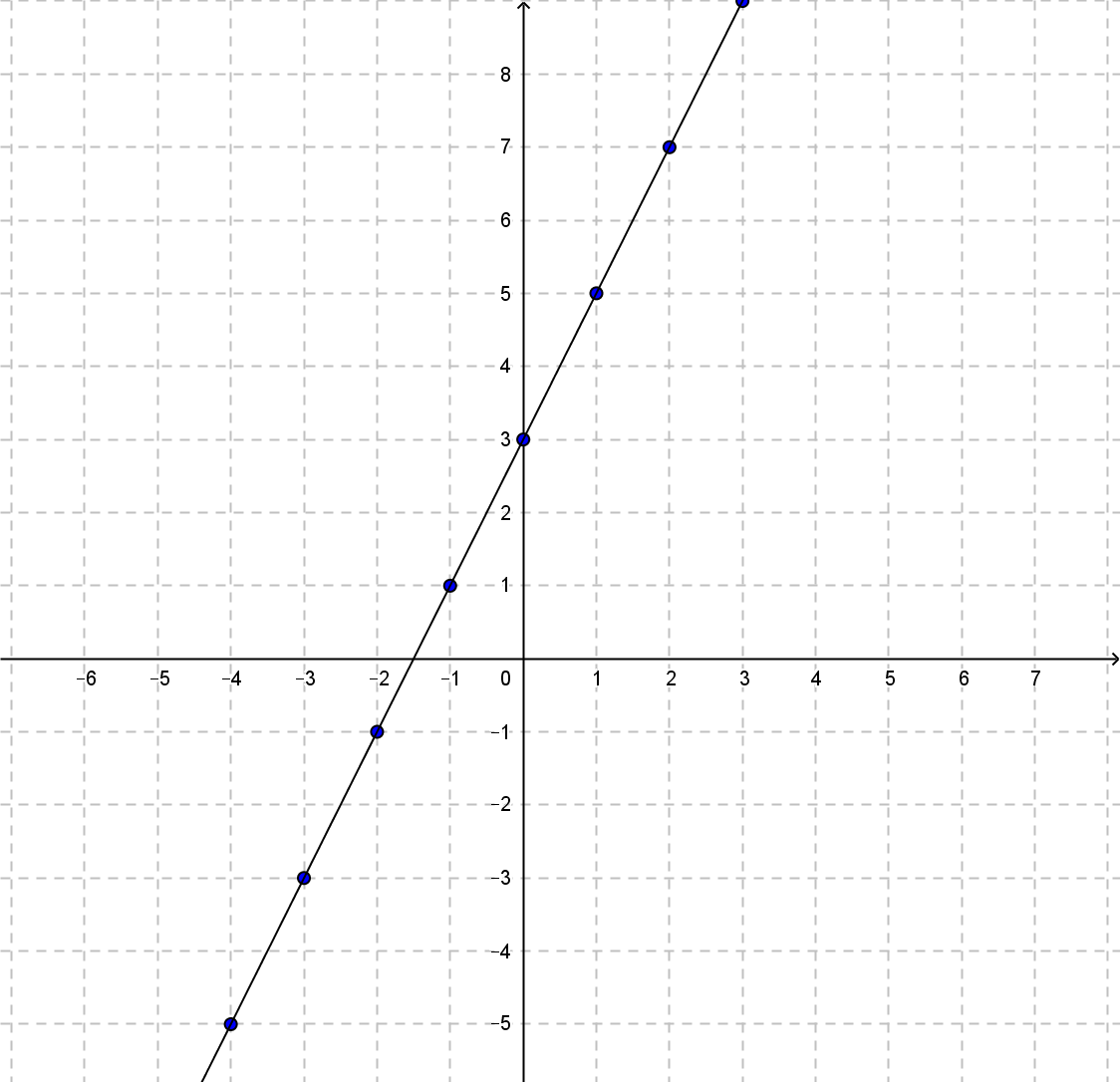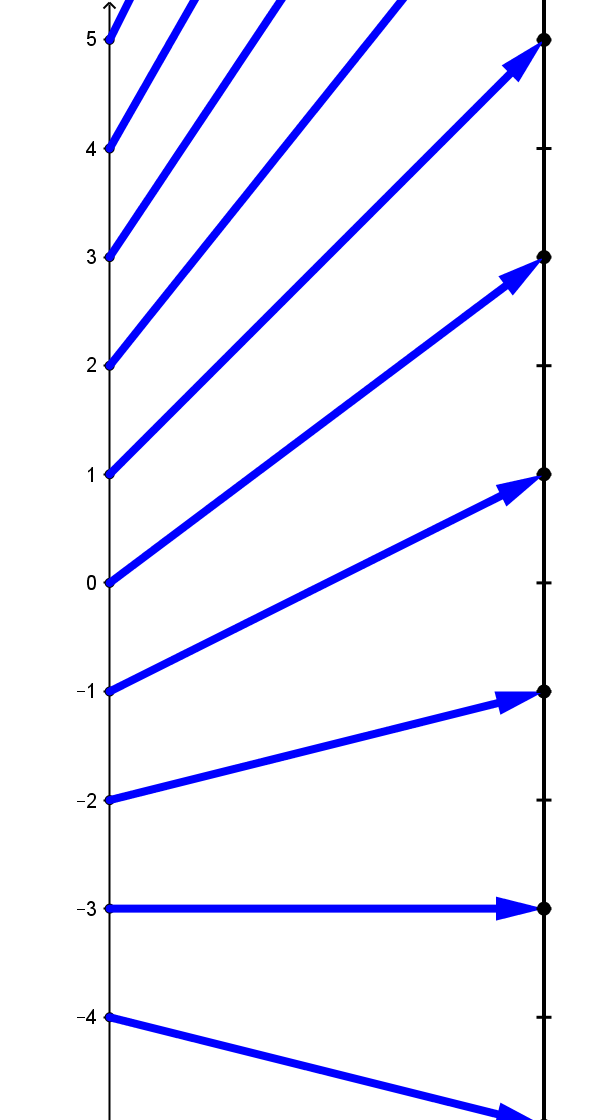Example LF.0:  Suppose \$y\$ is a function of \$x\$ given by the equation \$y = f (x) = 2x+ 3\$.
 \$x\$ \$f(x)=2x+3\$ 5 13 4 11 3 9 2 7 1 5 0 3 -1 1 -2 -1 -3 -3 -4 -5 -5 -7
Table LF1Graph LF1Diagram LF1

Table LF1 shows a selection of the values this function relates.

Graph LF1 shows the graph of the table values of the function \$f\$ as well as the visualization of the graph of (all the values of) the function for the interval domain \$[-5,5])\$.

Diagram LF1 shows the mapping diagram for most of the table values of the function \$f\$.